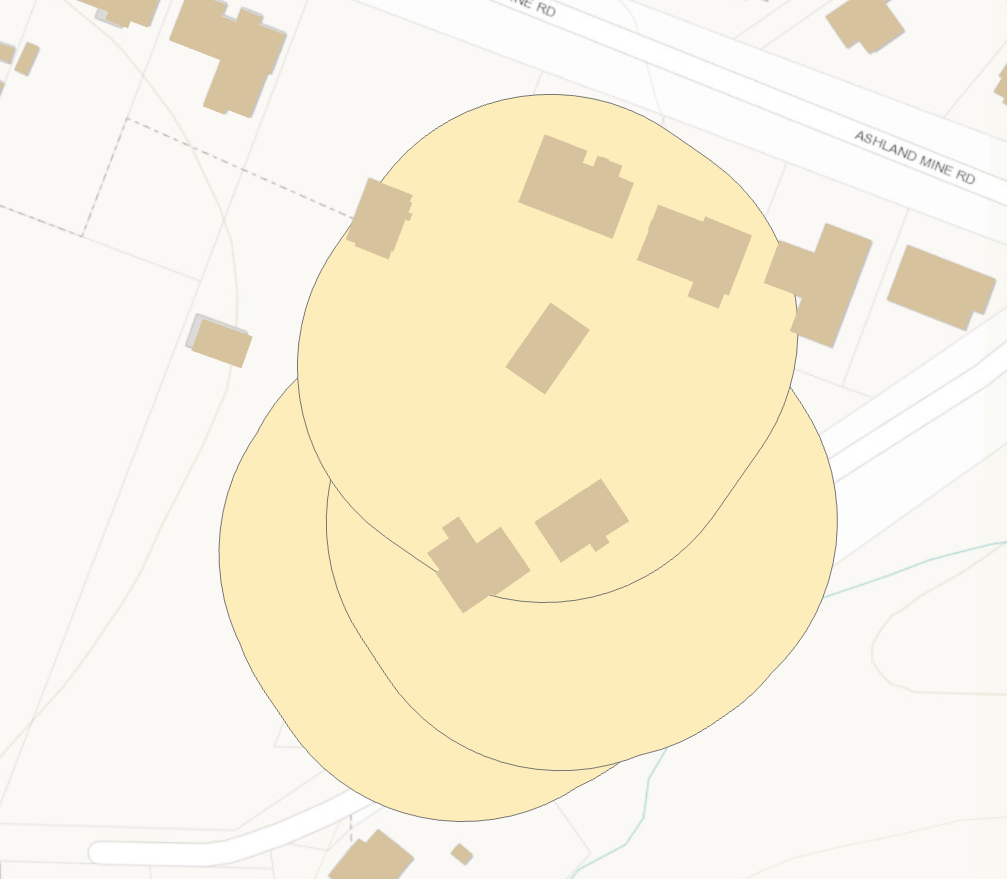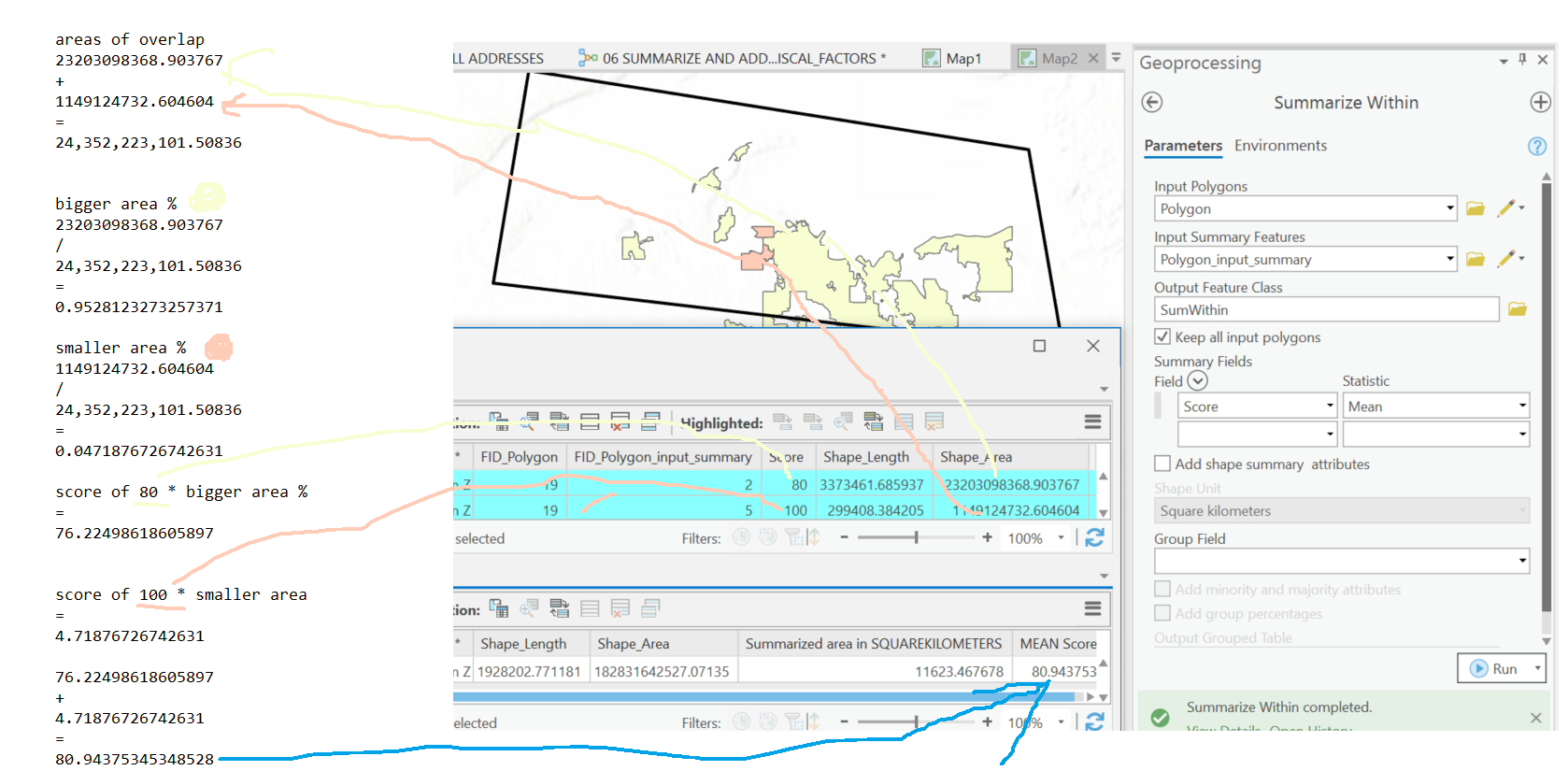# Arcgis Pro - Summarize Within

1921
9
03-12-2020 09:57 AMbyMVP Regular Contributor

I am trying to find out the average value of a nearby polygons using the Summarize within tool.

The problem I am running into is that the average is not what I expect it to be if I average it manually.

From the tool reference page Summarize Within—Help | Documentation

 in_polygons The polygons used to summarize the features, or portions of features, in the input summary layer.I think that it is using Portions of features within the 40 ft buffer I am using. I cannot find any documentation on when it uses a portion or all. I have tried getting the average of a polygon that has all of 4 buildings in it and it does not even get the average right when I would think that all the polygon would be used. At this point I don't know how it calculates "mean".

The page defines: Mean—Calculates the average of all the points in each polygon.

Any suggestions?

Tags (4)
1 Solution

Accepted SolutionsbyEsri Contributor

Dan Patterson‌ I just reviewed that tech support bug and the case logged is non repro in current Pro versions. The weighted mean computation appears correct. Here is the math I used for the black border polygon summarizing the two colored polygons inside it. The mean Score calculated for the output is based on the ratio of overlap between the two summary features. The yellow feature accounts for 95% of the total overlap, so the weighted score will be much closer to that value. The orange polygon has a higher value, but only accounts for ~5% of the overlap, so it just nudges the mean value up a little.9 RepliesbyMVP Esteemed Contributor

draw the polygon with no fill. I am wondering if the top polygon clips the building closest to its perimeter in the north end, which would leave you with one polygon that contains 2 buildings.byMVP Regular ContributorHere are the 4 values of the buildings that touch the polygon.

 35.3333 46.5 44.8333 38.6667

Here is the mean that the output results.

`43.646452`byMVP Esteemed ContributorbyMVP Regular Contributor

Dan Patterson‌ Well I guess I will look for a different way to do this...

Thanks for finding the tech support bug.byMVP Esteemed Contributor

I shall marked  as assumed answered thenbyEsri Contributor

Dan Patterson‌ I just reviewed that tech support bug and the case logged is non repro in current Pro versions. The weighted mean computation appears correct. Here is the math I used for the black border polygon summarizing the two colored polygons inside it. The mean Score calculated for the output is based on the ratio of overlap between the two summary features. The yellow feature accounts for 95% of the total overlap, so the weighted score will be much closer to that value. The orange polygon has a higher value, but only accounts for ~5% of the overlap, so it just nudges the mean value up a little.byMVP Esteemed Contributor

Ok that makes sense now, if they do indeed intersect the portion of the building that intersects with the circle.  The simple addition average you show would only be for buildings *completely within* the circle.byEsri Contributor

Hi Rickey Fite‌,

The mean is an area-weighted mean of those four buildings. The building that has the most overlap will contribute more towards the mean.

Based on your pictured values and the output mean value it is very hard to tell if the value is correct, there just isnt enough information. We need to know the area of overlap between the highlighted circle and each building that intersects it, then we weight those buildings values by how much of the total overlap they contribute. If you want me to double check the match and send you the results, send a sample dataset to dflater@esri.com

The mean WILL NOT be the average of (35.33333+46.5+44.83333+38.66667)/4. Doing an average like this throws away the spatial component of the analysis, since each of those buildings spatially overlaps the highlighted circle different.

If you want a simplified result that does the basic average I just described, Spatial Join can accomplish it using a MEAN Merge Rule. Spatial Join—Help | DocumentationbyMVP Regular Contributor

Thank you for getting back to me on this! it is very helpful. i might have uses for this in the future. I have now been told that this is not the calculation we want run.  I will be posting a new question and will link it here when its up.